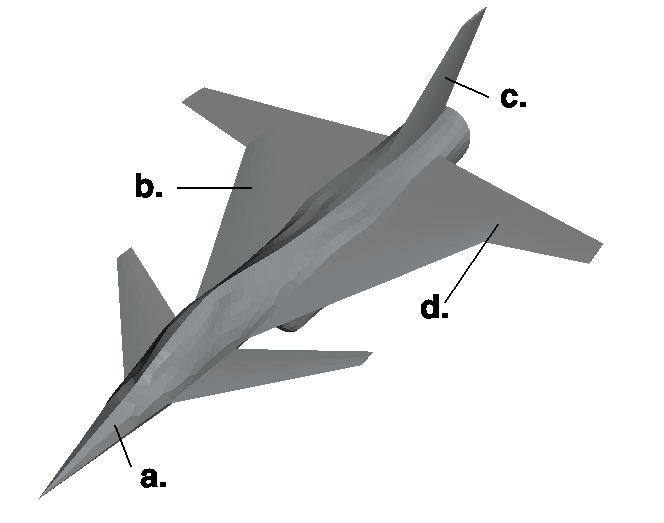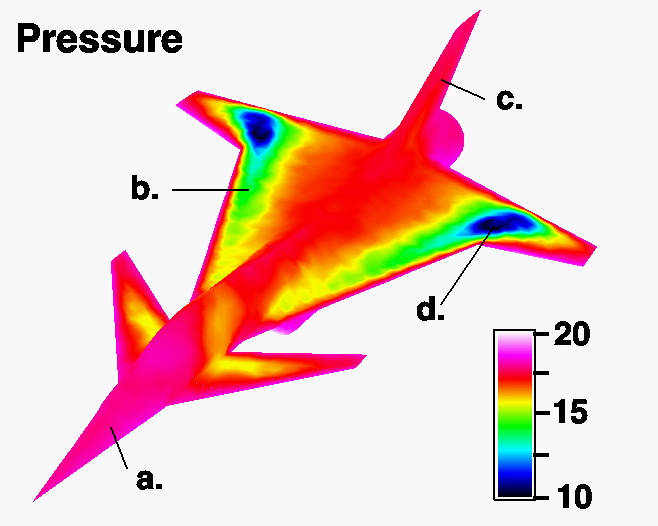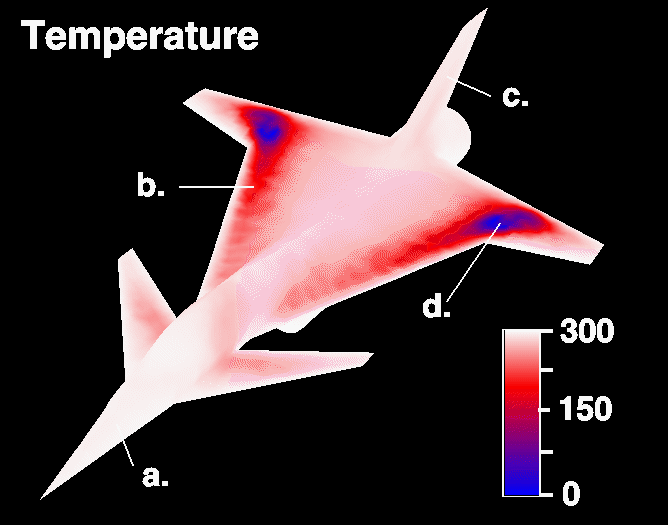PRINT THIS ANSWER SHEET TO FILL OUT AND TURN IN.

NAME_________________________________ CLASS____________________ DATE____________

## Temperature and Pressure Activities Worksheet

### Predict Temperature and Pressure1. Using the above diagram, rank the points on an airplane during flight from 1-4 (1=hottest, 4=coldest).

a. _______ b._______ c. ________ d. ________

2. Why do you believe your predictions are correct?

### Interpret Temperature and Pressure

Use the pressure diagram shown below to answer the following questions.

1. Determine the pressure at each of the four points.

a.___________ b.___________ c. ____________ d. ____________

2. What is the value of the notch on the chart between 20 and 15? ________________

3. What is the value of the notch on the chart between 15 and 10? ________________

4. What can you conclude from your observations of the airplane pressure points?Use the temperature diagram shown below to answer the following questions.

1.  Determine the temperature at each of the four points.

a.___________ b.___________ c. ____________ d. ____________

2. What is the value of the notch on the chart between 300 and 150? ________________

3. What is the value of the notch on the chart between 150 and 0? ________________

4. What can you conclude from your observations of the airplane temperature points?